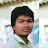Hour Calculator for work: Job Hours Tracker - Salary Calculator- Time CalculatorAPP Information

 Download Version 2.00 (7) Apk Size 3.25 MB App Developer TrasCo Studios Malware Check TRUSTED Install on Android 4.4.x and up App Package trasco.crist.calculadorajornada.apk MD5 a488c7ff103079de75a58b7ced32c944 Rate 5 Website https://x2c955a6e.app-ads-txt.com

## Download Hour Calculator for Work - Time Calc - Salary Calc 2.00 APK### App Description

```Hour Calculator for work: Job Hours Tracker - Salary Calculator- Time Calculator

Add,subtract,multiply or divide hours and minutes. Useful to calculate the job hours, salary or which work with better salary to choose.

Hour Calculator for work: Job Hours Tracker - Salary Calculator- Time Calculator: Calculate time difference between times. Store the records by date, calculate the total by days, weeks, months, hours and minutes.

Examples of this Hour Calculator for work: Job Hours Tracker - Salary Calculator- Time Calculator:

Hours and Minutes entry: 07:02
Hours and Minutes exit: 16:58
Time Calc Hour Calculator for work: 09:56
Price Hour: 11.57
Salary: 114.93

Hours and Minutes entry: 07:55
Hours and Minutes exit: 15:14
Time Calc Hour Calculator for work: 07:19
Price Hour: 9.9
Salary: 72.44

Controlling overtime in your work will be easier with this Hour Calculator for work: Job Hours Tracker - Salary Calculator- Time Calculator. You will be able to know the hours worked and the salary.

You can perform your calculations in hours, minutes or in decimal, assign them a price and calculate the salary.

Use the Hour Calculator for work: Job Hours Tracker - Salary Calculator- Time Calculator to set an hour of entry and exit, the Hour Calculator for work: Time Calculator - Salary Calculator- Job Hours Tracker will calculate it for you.

Perfect for workers to calculate in their work the Salary or Time Calc. With this Hour Calculator for work: Job Hours Tracker - Salary Calculator- Time Calculator you can calculate your salary easily.

This Hour Calculator for work: Job Hours Tracker - Salary Calculator- Time Calculator will also help us Time Calc between minutes and hours. See some example of calculations of hours and minutes:

Hour Calculator for work: Job Hours Tracker - Salary Calculator- Time Calculator:

Time Calc: 69 minutes +82 minutes +3:03 hours = 5:34 hours
Time Calc: 100 minutes +5:42 hours +72 minutes = 8:34 hours

It works well for part-time jobs and know at the end of the day the total hours worked and salary. We multiply it by the hour of work price and we will obtain the salary of the work.

Hour Calculator for work: Job Hours Tracker - Salary Calculator- Time Calculator: Do you know how many hours are 724 minutes? Time Calc: It's 12:04 hours. With this hour and minute calculator - Time Calc it is very easy. Do not waste time with calculations of complex hours, with this Hour Calculator for work: Job Hours Tracker - Salary Calculator- Time Calculator is easy.

It is useful to calculate hours or Time Calc in companies with many employees, we calculate the hours of an employee and know his salary. Do you have many hourly employees and don't know what salary they have? With this Hour Calculator for work: Job Hours Tracker - Salary Calculator- Time Calculator you will have it controlled.

This Hour Calculator for work: Job Hours Tracker - Salary Calculator- Time Calculator has many uses:

-Hour Calculator: Calculate Cumulative study hours.
-Salary calculator for my workers
-Hour Calculator: Calculate Hours I've lost in traffic jams.
-Hour Calculator that accumulate breaks at work.
-Hour Calculator: Calculate Hours and minutes of exercise per week.
-Salary calculator: Calculate the salary or salary of your different jobs.
-Hour Calculator: Calculate Hours and minutes I do in my work for hours.
-Salary calculator in my different jobs by hours.
-Hour Calculator: How long does it take to make a project.

With this Hour Calculator for work: Job Hours Tracker - Salary Calculator- Time Calculator you will not need to waste a minute in making all these difficult time calculations.

Try this Hour Calculator for work: Job Hours Tracker - Salary Calculator- Time Calculator make us a comment and we will improve this hours and minutes calculator for you!

Thank you for using this Hour Calculator for work: Job Hours Tracker - Salary Calculator- Time Calculator!
```

### App ChangeLog

• Improvement of functionalities: It allows modifying saved recordsB

### More Android Apps to Consider

1.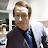Darren Marsh

Really good. Just something I would suggest to add is, a way of putting it in date order when adding more.

2.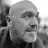Phil Simpson

Does the job well, I've tried a few similar apps but they don't seem to hit the mark as well as this one does. Trouble is I have yet to keep an app for long if I can't buy my out of adverts... there doesn't seem a way to do this so it's days are numbered unless the dev adds the option. It's just me... otherwise I can't fault it.

3.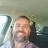Timothy Morrison

Super easy to use compared to other time calculators. Nice simple display and lay out.

4.Aaron Elkins

Basic time accounting doesn't apply to this. A shift from 1528h to 1829h is NOT 2.59h it's 2.98h. Time keeping is extremely important to have accurate. This can't even account for the difference of 60min vs .60 hours. They are not the same thing. Ugh.

5.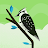panasa srujan

I would be better if it have Report pulling option through Pdf or Excel

6.Ruben Carrillo

This hour calculator for work is very useful to calculate the salary of work. I use this hour calculator for work to tracking hours work.

7.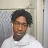the novastar

The app is good i love it i can get done with my work way more quicker then doin it with a number line im so happy thank you so much god bless you

8.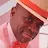Maurice E. Jones

Dull looking user interface; no features or customization

9.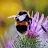Marc Lozano

Just what I was looking for. Thank you. Do you have a no ads version?

10.